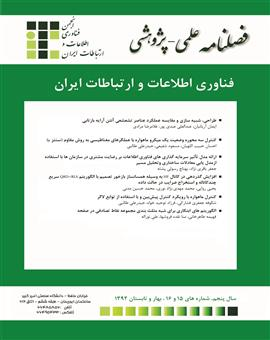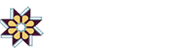•• #### List of Articles

• Open Access Article

1 - Heuristic algorithms for pseudo-triangulation random point set in the plane
mona naghdeforoshhs fahime taherkhani ali norollah
Finding algorithms for the diverse issues proposed in Computational Geometry including pseudo-triangulation of point set is among the scientific subjects, which has already attracted the attention of computer scientists'. A pseudo-triangle is a simple polygon in the pl Full Text
Finding algorithms for the diverse issues proposed in Computational Geometry including pseudo-triangulation of point set is among the scientific subjects, which has already attracted the attention of computer scientists'. A pseudo-triangle is a simple polygon in the plane with exactly three convex vertices, called corners. Three reflex chains of edges join the corners. A pseudo-triangulation for point set is a partition of the convex hull of point set into pseudo-triangles whose vertex set is point set. Many possible optimality criteria have been investigated for pseudo-triangulations which are often based on edge weights or angles in which computing a minimum weight pseudo-triangulation for a point set is among the open problems. A pseudo-triangulation is called minimum if it consists of exactly n-2 pseudo-triangles and the minimum number of edges needed for it is 2n-3. Every vertex of a minimum pseudo-triangulation is pointed. A vertex is pointed if it has an incident angle greater than π. With respect to the proposed cases, the aim of this thesis is to present new methods for pseudo-triangulation of point set in the plane, so that it can open new algorithmic reflections in this field. This research shows that the generation of convex hull layers for point set and their pseudo-triangulation, using two new algorithms which have been considered in this research, minimizes pseudo-triangulation. Also a new algorithm has been considered to generate spiral simple polygon such that the generation of random simple polygons has two main areas of application: testing the correctness and evaluating the CPU-time consumption of algorithms that operate on polygons Manuscript Document

### Rimag

Rimag is an integrated platform to accomplish all Scientific Journal requirements such as Submission, Evaluating and Reviewing, Editing, DOR assignment and publishing in the web.